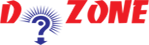﻿ What are Python Commands: Here is the list with examples## List of Python Commands

In a Python command, Comments start with the hash character "#" and it is extended to the end. A commnet may not appear within the string literal.

``````
For example:
print " Hello world " # this is the first comment
Hello World is not a Commment as it is inside the quotes.
```
```

Comments are not the part of command, but rather intended as documentation for anyone reading the code.

Multiline comments are also possible, and are enclosed by triple double-quote symbols:
``````
""" This is an example of a Multiline Comment
that goes on
and on
and on ."" "
```
```

### Numbers and Various other data types

Python recognizes several different types of data. For instance, 29 and −65 are integers, while 7.0 and −23.09 are floats or floating point numbers. The type float is (roughly) the same as a real number in mathematics. The number 12345678901 is a long integer ; Python prints it with an “L” appended to the end. Usually the type of a piece of data is determined implicitly.

#### The Type Function

To see the type of some data, use Python’s built-in type function:

``````
>>> type ( -65)
< type ’ int ’ >
>>> type (7.0)
< type ’ float ’>
>>> type (12345678901)
< type ’ long ’>
```
```

Another useful data type is complex, used for complex numbers.

``````
For example:
>>> 2j
2j
>>> 2j -1
( -1+2 j )
>>> complex (2 ,3)
(2+3 j)
>>> type ( -1+2 j)
< type ’ complex ’>
```
```

Notice that Python uses j for the complex unit (such that j2= −1) just as physicists do, instead of the letter i preferred by mathematicians.

```				```
Create Different Types of Lists:
# empty list
mylist = [ ]
# list of numbers
mylist = [2, 3, 4, 5]
#list with mixed datatypes
mylist = [2, “dzone”, 4.5]
# nested list
mylist = [“dzone”, [4, 6, 8], ['c']]
```
```

### Strings

Other useful data types are strings (short for “character strings”); for example "Hello World!".
Strings are sequences of characters enclosed in single or double quotes:

``````
>>> " This is a string "
’ This is a string ’
>>> ’ This is a string , too ’
’ This is a string , too ’
>>> type ( " This is a string ")
< type ’ str ’ >
```
```